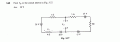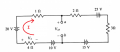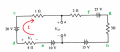# Assistance in solving for a voltage drop in a loop circuit (very rusty)

#### 10th&Bennett

Joined Apr 15, 2018
27
Greetings all -

First time poster here and I am very very rusty at Circuit theory. I am trying to get back into it, but am getting very frustrated at the same time. What may have been simple many years ago is just not clicking with me anymore.

I am working through problems in a Schaum's guide. I have attached the problem (diagram, Figure 3-27). They do provide the answer but not the steps. I am trying to find V_ab.

For this particular problem the answer is 20V. However, V_ab is in the middle of this circuit. The total resistance is 10 ohms, and the total voltage (I believe) is -35V (-20 +25 - 15 - 10), which would lead to a current of -3.5A. If signs didn't matter, then the total voltage would be 70V, and the current would be 7A. So that's my first clarification - which one is correct?

As for V_ab - the way I understand it is the V_ab is the voltage drop from node a to node b, which is the same of the voltage drops across the components connected between nodes a and b either to the right or left of node a.

I assume that we are doing KVL in some sense, where the sum of all voltages in a loop must equal zero. While I am not clear here either, and in doing both right and left loops with both -3.5 A and 7A, I do not get 20V for V_ab.

I am trying to understand where I am going wrong so I can get better at this. Any guidance would be helpful.

Thanks!
10th

Mod: Added clip from PDF.#### Attachments

• 491.3 KB Views: 6
Last edited by a moderator:

#### ericgibbs

Joined Jan 29, 2010
16,031
hi 10th
Welcome to AAC.
Is this self study or a homework assignment.?
E

#### 10th&Bennett

Joined Apr 15, 2018
27
Thanks for the response. Self-study. Graduated many moons ago!

#### 10th&Bennett

Joined Apr 15, 2018
27

#### ericgibbs

Joined Jan 29, 2010
16,031
hi,
Check the polarity of your V2.
E

#### 10th&Bennett

Joined Apr 15, 2018
27
I just saw your links @ericgibbs - Ok, but can he hold off on Norton's for now? That's next but I want to get the fundamentals down first before I move on to that.

#### 10th&Bennett

Joined Apr 15, 2018
27
Oh ok I'll repost with a another figure.

#### WBahn

Joined Mar 31, 2012
26,928
First and foremost, signs almost always matter (the 'almost' being little more than a sheet anchor).

I also don't know how you get that (-20 +25 - 15 - 10) comes out to be -35 V.

First thing you need to do is pick a direction for current in the loop. It doesn't matter which direction, clockwise or counterclockwise, but pick one.

I generally pick clockwise.Then simply write the KVL equation in the direction of the current, summing either the voltage gains or the voltage drops and setting the result equal to zero. I generally sum the voltage drops. Thus the voltage drop going across a resistor is the current multiplied by the resistance while the voltage drop going from the positive terminal of a source to the negative terminal is the source voltage while going from the negative terminal to the positive terminal it is the negative of the source voltage.

So starting from the node 'b', we have:

(-10 V) + I(4 Ω) + (-20 V) + I(1 Ω) + I(2 Ω) + (25 V) + I(3 Ω) + (-15 V) = 0

No solve for the current I.

Once you have that, you can calculate Vab walking across any path from 'a' to 'b' and summing up the voltage drops along that path (or from 'b' to 'a' summing up the voltage gains).

#### 10th&Bennett

Joined Apr 15, 2018
27
So I changed the polarities but I thought the old way for 10V was correct.

#### 10th&Bennett

Joined Apr 15, 2018
27
@WBahn thanks for the clarification. Don't mean to try your patience but I stated I was very rusty.

Question, why did you do KVL on the "big loop"? To me I saw it as two loops since V_ab is in the middle.

Thanks again. I will try your approach.

#### ericgibbs

Joined Jan 29, 2010
16,031
hi 10,
Consider the 15V and 25V sources are opposing polarity so how can the sum be 35V.?

E

#### WBahn

Joined Mar 31, 2012
26,928
So I changed the polarities but I thought the old way for 10V was correct.
The problems you seem to be having have more to do with arithmetic that circuit theory. So until you blow that rust off, be sure to spend extra time double and triple checking everything you do arithmetically.

One thing that can help is to ask 'sanity check' questions as you go.

Does the 25 V source tend to make Node 'a' higher or lower voltage than Node 'b'? (Answer: Node a)

Does the 15 V course tend to make Node 'a' higher or lower voltage than Node 'b'? (Answer: Node b)

Are the 25 V and 15 V sources connected so that they oppose each other, or reinforce each other? (Answer: Oppose)

Since they oppose each other, the net voltage will have a magnitude of the difference, or 10 V.

Since the stronger of the two (the 25 V source) tends to make Node 'a' more positive, the net result will still tend to make Node 'a' more positive.

So your equivalent source should have a magnitude of 10 V and be connected in such a way that it tends to make Node 'a' higher than Node 'b'.

If what you come up with doesn't meet this sanity check, then something is wrong.

#### 10th&Bennett

Joined Apr 15, 2018
27
@ericgibbs - regarding polarity (and I want to be solid here). So my line of thinking on the left side was that since they are same polarities they can combine 20+10 = 30 V. On the right side since they are different polarities I thought it was 25-15=10V originally.

As far as the -35V goes, I honestly thought I did the KVL Loop with voltages, so going clockwise -20V + 25V - 15V - 10V = -35V. I thought that since I was going clockwise the minus polarity (the short bar) meant the voltage was negative across the DC source, and when you hit the positive source it would go positive.

#### WBahn

Joined Mar 31, 2012
26,928
@WBahn thanks for the clarification. Don't mean to try your patience but I stated I was very rusty.

Question, why did you do KVL on the "big loop"? To me I saw it as two loops since V_ab is in the middle.

Thanks again. I will try your approach.
You can certainly set it up as two loops, meaning that you have two unknown currents to solve for. But in that case both loops include a segment that has infinite resistance. If the two loop currents are defined to be in the same direction, that then means that they have to be identical so that, across that open gap, they exactly cancel out. When you apply that constraint, the net result is the exact same equation you get by applying a single loop equation to the outer loop.

#### WBahn

Joined Mar 31, 2012
26,928
@ericgibbs - regarding polarity (and I want to be solid here). So my line of thinking on the left side was that since they are same polarities they can combine 20+10 = 30 V. On the right side since they are different polarities I thought it was 25-15=10V originally.

As far as the -35V goes, I honestly thought I did the KVL Loop with voltages, so going clockwise -20V + 25V - 15V - 10V = -35V. I thought that since I was going clockwise the minus polarity (the short bar) meant the voltage was negative across the DC source, and when you hit the positive source it would go positive.
Again, how does -20V + 25V - 15V - 10V = -35V ???

Do you see why I say that your biggest problem, right now, is arithmetic and not circuit theory?

There is no way that you can add or subtract those four magnitudes and end up with an odd value.

#### 10th&Bennett

Joined Apr 15, 2018
27
Oh crap! What was I thinking!?!?! Ok fair fair I'll own that. -20V.

Quick question, and I don't know if there's a shortcut (and I'm not advocating this approach, but genuinely curious), but when you look at the circuit and you add up the DC voltages, can you just say V_ab is approximately 20V due to adding the sources?

#### WBahn

Joined Mar 31, 2012
26,928
Oh crap! What was I thinking!?!?! Ok fair fair I'll own that. -20V.

Quick question, and I don't know if there's a shortcut (and I'm not advocating this approach, but genuinely curious), but when you look at the circuit and you add up the DC voltages, can you just say V_ab is approximately 20V due to adding the sources?
No. But asking questions like this is a very good way to improve your understanding, whether you end up being right or wrong (in fact, the wrong ones tend to teach you more).

First off, -20 V and +20 V are not the same. Not even close. They differ by 40 V. Don't let things that, have the same magnitude lull you into thinking that they are the same thing.

But let's explore your question in terms of sanity check considerations.

What you are proposing is that the voltage across ANY two nodes in the circuit is going to be approximately equal to the sum of the DC voltages around the entire circuit. That has to be what you are claiming since 'a' and 'b' are just two arbitrary nodes in the circuit and the person that created the problem could have chosen any two nodes as 'a' and 'b'.

What if they have chosen 'a' to be the positive terminal of the 10 V source and left 'b' where it is? Then V_ab would clearly have been 10 V.

What if they had chosen 'a' to be the negative terminal of the 15 V source and left 'b' where it is? Then V_ab would clearly have been -15 V.

#### 10th&Bennett

Joined Apr 15, 2018
27
Ah thank you @WBahn for the warning on the magnitude. To be clear I was not advocating a shortcut, but again just curious.

So, I did go back to the "big loop" approach you did above. The current per the KVL equation you wrote is 2 Amps.

You stated, "You can calculate Vab walking across any path from 'a' to 'b' and summing up the voltage drops along that path (or from 'b' to 'a' summing up the voltage gains.

Confirming - The "walking approach" path from a-to-b would be 2 ohms(2Amps) + 25 V + (3ohms*2amps) - 15 V = 20V.

Now, from b to a it would be -10V + 4(2) - 20V +1(2) = -20V however (and correct me if I am wrong here because I want to be solid on this) the voltages should be positive since the b terminal is negative, and since you're going in a clockwise direction the voltage drops on that side would be subtracted and the voltages added. It would come out to be 20 V then in line with the answer.

a-to-b path I am fairly confident on. B-to-a, I would just have verification.

Thanks again for your patience. The bulb is starting to come back on but still dim!

#### WBahn

Joined Mar 31, 2012
26,928
Ah thank you @WBahn for the warning on the magnitude. To be clear I was not advocating a shortcut, but again just curious.

So, I did go back to the "big loop" approach you did above. The current per the KVL equation you wrote is 2 Amps.

You stated, "You can calculate Vab walking across any path from 'a' to 'b' and summing up the voltage drops along that path (or from 'b' to 'a' summing up the voltage gains.

Confirming - The "walking approach" path from a-to-b would be 2 ohms(2Amps) + 25 V + (3ohms*2amps) - 15 V = 20V.

Now, from b to a it would be -10V + 4(2) - 20V +1(2) = -20V however (and correct me if I am wrong here because I want to be solid on this) the voltages should be positive since the b terminal is negative, and since you're going in a clockwise direction the voltage drops on that side would be subtracted and the voltages added. It would come out to be 20 V then in line with the answer.

a-to-b path I am fairly confident on. B-to-a, I would just have verification.

Thanks again for your patience. The bulb is starting to come back on but still dim!
In going from 'b' to 'a', you need to sum up the voltage GAINS.

So V_ab = (+10 V) - (4 Ω)(2 A) + (20 V) - (1 Ω)(2 A) = +20 V

A super explicit way to approach it is using double-subscript notation math.

V_ab = (V_a - Vb)

Thus, if I have some node, call it 'c', between Node 'a' and Node 'b' I can write:

V_ab = (V_a - V_b) + (V_c - V_c)

Notice that all I am doing here is adding zero to the right hand side, which changes nothing. But now I can rearrange things to get

V_ab = (V_a - V_c) + (V_c - V_b)

Now I can use the double-subscript notation to write this as

V_ab = V_ac + V_cb

So let's assign a label to every node and then write V_ab in terms of voltage differences across individual components.Going the left side, we have:

V_ab = V_ac + V_cd + V_de + V_eb

Going the right side, we have:

V_ab = V_af + V_fg + V_gh + V_hb

Now we can write these individual voltage differences with pretty high confidence:

V_ac = -I·(1 Ω) = -(2 A)(1 Ω) = -2 V
V_cd = 20 V
V_de = -I·(4 Ω) = -(2 A)(4 Ω) = -8 V
V_eb = 10 V
V_af = I·(2 Ω) = (2 A)(2 Ω) = 4 V
V_fg = 25 V
V_gh = I·(3 Ω) = (2 A)(3 Ω) = 6 V
V_hb = -15 V

V_ab = V_ac + V_cd + V_de + V_eb = (-2 V) + (20 V) + (-8 V) + (10 V) = 20 V

V_ab = V_af + V_fg + V_gh + V_hb = (4 V) + (25 V) + (6 V) + (-15 V) = 20 V

On a separate note, you want to start tracking your units explicitly throughout your work. When you have:

-10V + 4(2) - 20V +1(2) = -20V

You have a voltage added to a pure number added to a voltage added to a pure number that somehow yields a voltage at the end.

Physical quantities have units and those units matter. Most (not all) mistakes you make will mess up the units, but only if the units are there to be messed up. By tracking your units and checking, at every step of your work, whether the units are still working out properly, you will catch most of your silly mistakes (which we ALL make on a frequent basis) almost immediately, saving you much time, effort, and grief in the long run. In just a short amount of time it will become so natural that you won't even realize you are doing it.